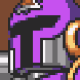# Wormhole

``````shader_type spatial;
render_mode depth_draw_alpha_prepass, cull_front, specular_schlick_ggx, depth_test_disable, ensure_correct_normals, shadows_disabled, ambient_light_disabled;

uniform sampler2D tex_frg_32;
uniform sampler2D tex_frg_3;
uniform vec4 MainColor : hint_color;
uniform vec4 BackColor1 : hint_color;
uniform vec4 BackColor2 : hint_color;
uniform float Brightness;

void vertex() {
// Input:8
float n_out8p0 = TIME;

// Input:2
vec3 n_out2p0 = VERTEX;

// VectorDecompose:3
float n_out3p0 = n_out2p0.x;
float n_out3p1 = n_out2p0.y;
float n_out3p2 = n_out2p0.z;

// ScalarOp:7
float n_out7p0 = n_out8p0 + n_out3p1;

// ScalarOp:14
float n_in14p1 = 3.00000;
float n_out14p0 = n_out7p0 * n_in14p1;

// ScalarFunc:5
float n_out5p0 = sin(n_out14p0);

// ScalarOp:13
float n_in13p1 = 0.10000;
float n_out13p0 = n_out5p0 * n_in13p1;

// ScalarOp:6
float n_out6p0 = n_out13p0 + n_out3p0;

// ScalarOp:9
float n_out9p0 = n_out8p0 + n_out3p0;

// ScalarOp:15
float n_in15p1 = 1.00000;
float n_out15p0 = n_out9p0 * n_in15p1;

// ScalarFunc:11
float n_out11p0 = cos(n_out15p0);

// ScalarOp:12
float n_in12p1 = 0.20000;
float n_out12p0 = n_out11p0 * n_in12p1;

// ScalarOp:10
float n_out10p0 = n_out12p0 + n_out3p2;

// VectorCompose:4
vec3 n_out4p0 = vec3(n_out6p0, n_out3p1, n_out10p0);

// Output:0
VERTEX = n_out4p0;

}

void fragment() {
// Input:2
vec3 n_out2p0 = vec3(UV, 0.0);

// VectorDecompose:9
float n_out9p0 = n_out2p0.x;
float n_out9p1 = n_out2p0.y;
float n_out9p2 = n_out2p0.z;

// ScalarSmoothStep:20
float n_in20p0 = 0.00000;
float n_in20p1 = 0.20000;
float n_out20p0 = smoothstep(n_in20p0, n_in20p1, n_out9p1);

// Input:34
float n_out34p0 = TIME;

// ScalarOp:35
float n_in35p1 = -0.20000;
float n_out35p0 = n_out34p0 * n_in35p1;

// ScalarOp:36
float n_in36p1 = -0.30000;
float n_out36p0 = n_out34p0 * n_in36p1;

// VectorCompose:37
float n_in37p2 = 0.00000;
vec3 n_out37p0 = vec3(n_out35p0, n_out36p0, n_in37p2);

// VectorOp:38
vec3 n_out38p0 = n_out37p0 + n_out2p0;

// VectorOp:53
vec3 n_in53p1 = vec3(1.00000, 4.00000, 1.00000);
vec3 n_out53p0 = n_out38p0 * n_in53p1;

// Texture:32

// ScalarOp:54
float n_out54p0 = n_out20p0 - dot(n_out32p0, vec3(0.333333, 0.333333, 0.333333));

// ScalarOp:56
float n_out56p0 = n_out54p0 + n_out9p1;

// ScalarClamp:52
float n_in52p1 = 0.00000;
float n_in52p2 = 1.00000;
float n_out52p0 = clamp(n_out56p0, n_in52p1, n_in52p2);

// Input:60
vec3 n_out60p0 = vec3(SCREEN_UV, 0.0);

// Input:6
float n_out6p0 = TIME;

// ScalarOp:7
float n_in7p1 = 0.10000;
float n_out7p0 = n_out6p0 * n_in7p1;

// ScalarOp:8
float n_in8p1 = -0.20000;
float n_out8p0 = n_out6p0 * n_in8p1;

// VectorCompose:5
float n_in5p2 = 0.00000;
vec3 n_out5p0 = vec3(n_out7p0, n_out8p0, n_in5p2);

// VectorOp:4
vec3 n_out4p0 = n_out5p0 + n_out2p0;

// Texture:3

// VectorOp:33
vec3 n_out33p0 = n_out32p0 * n_out3p0;

// ScalarSmoothStep:70
float n_in70p0 = 0.20000;
float n_in70p1 = 0.80000;
float n_out70p0 = smoothstep(n_in70p0, n_in70p1, n_out9p1);

// VectorOp:66
vec3 n_out66p0 = n_out33p0 * vec3(n_out70p0);

// VectorOp:65
vec3 n_out65p0 = n_out60p0 + n_out66p0;

// Input:61
vec3 n_out61p0 = NORMAL;

// Fresnel:62
float n_in62p3 = 1.00000;
float n_out62p0 = pow(1.0 - clamp(dot(NORMAL, VIEW), 0.0, 1.0), n_in62p3);

// VectorScalarMix:63
vec3 n_out63p0 = mix(n_out65p0, n_out61p0, n_out62p0);

// Input:58

// Texture:59
vec3 n_out59p0;
float n_out59p1;
{
}

// ColorUniform:80
vec3 n_out80p0 = MainColor.rgb;
float n_out80p1 = MainColor.a;

// ColorUniform:78
vec3 n_out78p0 = BackColor1.rgb;
float n_out78p1 = BackColor1.a;

// ColorUniform:79
vec3 n_out79p0 = BackColor2.rgb;
float n_out79p1 = BackColor2.a;

// Input:72
float n_out72p0 = TIME;

// ScalarFunc:71
float n_out71p0 = sin(n_out72p0);

// ScalarOp:74
float n_in74p1 = 1.00000;
float n_out74p0 = n_out71p0 + n_in74p1;

// ScalarOp:75
float n_in75p1 = 2.00000;
float n_out75p0 = n_out74p0 / n_in75p1;

// VectorScalarMix:73
vec3 n_out73p0 = mix(n_out78p0, n_out79p0, n_out75p0);

// VectorScalarMix:49
vec3 n_out49p0 = mix(n_out80p0, n_out73p0, n_out9p1);

// ScalarSmoothStep:46
float n_in46p0 = 1.00000;
float n_in46p1 = 0.00000;
float n_out46p0 = smoothstep(n_in46p0, n_in46p1, n_out9p1);

// VectorScalarMix:27
vec3 n_in27p1 = vec3(0.00000, 0.00000, 0.00000);
vec3 n_out27p0 = mix(n_out49p0, n_in27p1, n_out46p0);

// Color:69
vec3 n_out69p0 = vec3(0.025314, 0.034936, 0.137276);
float n_out69p1 = 1.000000;

// ScalarOp:67
float n_out67p0 = dot(n_out33p0, vec3(0.333333, 0.333333, 0.333333)) * n_out46p0;

// VectorScalarMix:68
vec3 n_out68p0 = mix(n_out27p0, n_out69p0, n_out67p0);

// ScalarUniform:77
float n_out77p0 = Brightness;

// VectorOp:51
vec3 n_out51p0 = n_out68p0 * vec3(n_out77p0);

// VectorScalarMix:64
vec3 n_out64p0 = mix(n_out59p0, n_out51p0, n_out62p0);

// Output:0
ALPHA = n_out52p0;
EMISSION = n_out64p0;

}

void light() {
// Output:0

}
``````
###### Tags
portal, space, WormholeThe shader code and all code snippets in this post are under CC0 license and can be used freely without the author's permission. Images and videos, and assets depicted in those, do not fall under this license. For more info, see our License terms.
Subscribe
Notify ofInline Feedbacks1 year ago

Could you provide some context (or maybe a demo project) as to how to use this shader? I tried making a MeshInstance with a PlaneMesh, adding a new ShaderMaterial and selecting this shader, but I don’t see anything in the 3D view.1 year ago

Using a SphereMesh I can see the shape, but it always looks white, even if I change the colors in the params.DuckDuckGoosee3
1 month ago

I know this was a while ago and probably not being updated, but I wanted to use this with a game I’m making, but it wouldn’t work, I saw the comment and tried to download the repository, but when I use it in Godot 4, it says it is for Godot 3.

Normally this isn’t an issue, I just installed Godot 3. But, when I ran it, it said “project.godot” was misconfigured or may be missing. I checked: it wasn’t missing.

I don’t know what that means but upon examination,
“Shader-playground/project.godot: config_version = 4,” and I’m guessing (just guessing) that is the issue.

I’m not sure if you’re active here, so I will also be posting this on the repository to try to reach you.

Edit: I am unable to post this on the repository because google verification hates me 😀

Edit 2x: Really hope you see this lol

Last edited 1 month ago by DuckDuckGoosee3ColorauGuiyino
1 year ago